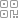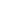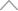6 5 1 3 9 2 6

# 1 IMU与轮速计的简介

## 1.1 IMU

### 1.1.1 简介

IMU全称Inertial Measurement Unit，惯性测量单元，主要用来检测和测量加速度与旋转运动的传感器。

IMU一般包括3个功能，

• 3轴加速度计：测量 x y z 3个方向上的加速度，z轴的加速度也就是重力值的大小(IMU水平的情况下)

• 3轴陀螺仪(角度度计)：测量出IMU分别绕着 x y z 轴旋转的角速度

• 3轴磁力计：测出地球磁场的方向，用于确定IMU 在 x y z 轴的方向，但是受磁铁，金属等影响较大．

IMU的频率可以很高，10hz-400hz 不等．

### 1.1.2 ros中的消息格式

IMU在ROS中的消息格式如下，有些IMU会提供积分后的姿态值，也就是orientation，有些不会提供，就需要自己通过对角速度进行积分来获取姿态值．

\$ rosmsg show sensor_msgs/Imu

uint32 seq

time stamp # 时间戳

string frame_id # 坐标系

geometry_msgs/Quaternion orientation # IMU的当前姿态，4元数的形式表示

float64 x

float64 y

float64 z

float64 w

float64 orientation_covariance # 姿态的协方差

geometry_msgs/Vector3 angular_velocity # IMU的3轴角速度

float64 x

float64 y

float64 z

float64 angular_velocity_covariance # IMU的3轴角速度的协方差

geometry_msgs/Vector3 linear_acceleration # IMU的3轴加速度

float64 x

float64 y

float64 z

float64 linear_acceleration_covariance # IMU的3轴加速度的协方差

## 1.2 轮速计

### 1.2.2 ros中的消息格式

\$ rosmsg show nav_msgs/Odometry

uint32 seq

time stamp # 时间戳

string frame_id # 里程计坐标系名字，一般为odom

string child_frame_id # 里程计坐标系指向的子坐标系名字，一般为base_link或者footprint

geometry_msgs/PoseWithCovariance pose # 带协方差的位姿

geometry_msgs/Pose pose

geometry_msgs/Point position #　位置，x y z

float64 x

float64 y

float64 z

geometry_msgs/Quaternion orientation #　四元数表示的姿态

float64 x

float64 y

float64 z

float64 w

float64 covariance

geometry_msgs/TwistWithCovariance twist # 带协方差的速度值

geometry_msgs/Twist twist

geometry_msgs/Vector3 linear #　线速度

float64 x

float64 y

float64 z

geometry_msgs/Vector3 angular #　角速度

float64 x

float64 y

float64 z

float64 covariance

# 2 代码讲解

## 2.1 获取代码

https://github.com/xiangli0608/Creating-2D-laser-slam-from-scratch

Creating-2D-laser-slam-from-scratch/lesson5/src/lidar_undistortion.cc

Creating-2D-laser-slam-from-scratch/lesson5/include/lesson5/lidar_undistortion.h

## 2.2 回调函数

// imu的回调函数

void LidarUndistortion::ImuCallback(const sensor_msgs::Imu::ConstPtr &imuMsg)

{

std::lock_guard<std::mutex> lock(imu_lock_);

imu_queue_.push_back(*imuMsg);

}

// odom的回调函数

void LidarUndistortion::OdomCallback(const nav_msgs::Odometry::ConstPtr &odometryMsg)

{

std::lock_guard<std::mutex> lock(odom_lock_);

odom_queue_.push_back(*odometryMsg);

}

// scan的回调函数

void LidarUndistortion::ScanCallback(const sensor_msgs::LaserScan::ConstPtr &laserScanMsg)

{

// 缓存雷达数据

if (!CacheLaserScan(laserScanMsg))

return;

// 如果使用imu，就对imu的数据进行修剪，进行时间同步，并计算雷达数据时间内的旋转

if (use_imu_)

{

if (!PruneImuDeque())

return;

}

// 如果使用odom，就对odom的数据进行修剪，进行时间同步，并计算雷达数据时间内的平移

if (use_odom_)

{

if (!PruneOdomDeque())

return;

}

// 对雷达数据的每一个点进行畸变的去除

CorrectLaserScan();

// 将较正过的雷达数据以PointCloud2的形式发布出去

PublishCorrectedPointCloud();

// 参数重置

ResetParameters();

}

## 2.3 缓存雷达数据

// 缓存雷达数据

bool LidarUndistortion::CacheLaserScan(const sensor_msgs::LaserScan::ConstPtr &laserScanMsg)

{

if (first_scan_)

{

first_scan_ = false;

// 雷达数据间的角度是固定的，因此可以将对应角度的cos与sin值缓存下来，不用每次都计算

CreateAngleCache(laserScanMsg);

scan_count_ = laserScanMsg->ranges.size();

}

corrected_pointcloud_->points.resize(laserScanMsg->ranges.size());

// 缓存雷达数据

laser_queue_.push_back(*laserScanMsg);

// 缓存两帧雷达数据，以防止imu或者odom的数据不能包含雷达数据

if (laser_queue_.size() < 2)

return false;

// 取出队列中的第一个数据

current_laserscan_ = laser_queue_.front();

laser_queue_.pop_front();

// 获取这帧雷达数据的起始，结束时间

current_scan_time_increment_ = current_laserscan_.time_increment;

current_scan_time_end_ = current_scan_time_start_ + current_scan_time_increment_ * (scan_count_ - 1);

return true;

}

## 2.4 IMU的时间同步与角度积分

// 修剪imu队列，以获取包含 当前帧雷达时间 的imu数据及转角

bool LidarUndistortion::PruneImuDeque()

{

std::lock_guard<std::mutex> lock(imu_lock_);

// imu数据队列的头尾的时间戳要在雷达数据的时间段外

if (imu_queue_.empty() ||

imu_queue_.front().header.stamp.toSec() > current_scan_time_start_ ||

{

ROS_WARN("Waiting for IMU data ...");

return false;

}

// 修剪imu的数据队列，直到imu的时间接近这帧点云的时间

while (!imu_queue_.empty())

{

if (imu_queue_.front().header.stamp.toSec() < current_scan_time_start_ - 0.1)

imu_queue_.pop_front();

else

break;

}

if (imu_queue_.empty())

return false;

current_imu_index_ = 0;

sensor_msgs::Imu tmp_imu_msg;

double current_imu_time, time_diff;

for (int i = 0; i < (int)imu_queue_.size(); i++)

{

tmp_imu_msg = imu_queue_[i];

if (current_imu_time < current_scan_time_start_)

{

// 初始角度为0

if (current_imu_index_ == 0)

{

imu_rot_x_ = 0;

imu_rot_y_ = 0;

imu_rot_z_ = 0;

imu_time_ = current_imu_time;

++current_imu_index_;

}

continue;

}

// imu时间比雷达结束时间晚，就退出

if (current_imu_time > current_scan_time_end_)

break;

// get angular velocity

double angular_x, angular_y, angular_z;

angular_x = tmp_imu_msg.angular_velocity.x;

angular_y = tmp_imu_msg.angular_velocity.y;

angular_z = tmp_imu_msg.angular_velocity.z;

// 对imu的角速度进行积分，当前帧的角度 = 上一帧的角度 + 当前帧角速度 * (当前帧imu的时间 - 上一帧imu的时间)

double time_diff = current_imu_time - imu_time_[current_imu_index_ - 1];

imu_rot_x_[current_imu_index_] = imu_rot_x_[current_imu_index_ - 1] + angular_x * time_diff;

imu_rot_y_[current_imu_index_] = imu_rot_y_[current_imu_index_ - 1] + angular_y * time_diff;

imu_rot_z_[current_imu_index_] = imu_rot_z_[current_imu_index_ - 1] + angular_z * time_diff;

imu_time_[current_imu_index_] = current_imu_time;

++current_imu_index_;

}

// 对current_imu_index_进行-1操作后，current_imu_index_指向当前雷达时间内的最后一个imu数据

--current_imu_index_;

return true;

}

## 2.5 轮速计的时间同步与平移计算

// 修剪imu队列，以获取包含 当前帧雷达时间 的odom的平移距离

bool LidarUndistortion::PruneOdomDeque()

{

std::lock_guard<std::mutex> lock(odom_lock_);

// imu数据队列的头尾的时间戳要在雷达数据的时间段外

if (odom_queue_.empty() ||

odom_queue_.front().header.stamp.toSec() > current_scan_time_start_ ||

{

ROS_WARN("Waiting for Odometry data ...");

return false;

}

// 修剪odom的数据队列，直到odom的时间接近这帧点云的时间

while (!odom_queue_.empty())

{

if (odom_queue_.front().header.stamp.toSec() < current_scan_time_start_ - 0.1)

odom_queue_.pop_front();

else

break;

}

if (odom_queue_.empty())

return false;

// get start odometry at the beinning of the scan

double current_odom_time;

for (int i = 0; i < (int)odom_queue_.size(); i++)

{

if (current_odom_time < current_scan_time_start_)

{

start_odom_msg_ = odom_queue_[i];

continue;

}

if (current_odom_time <= current_scan_time_end_)

{

end_odom_msg_ = odom_queue_[i];

}

else

break;

}

// if (int(round(start_odom_msg_.pose.covariance)) != int(round(end_odom_msg_.pose.covariance)))

// return false;

tf::Quaternion orientation;

double roll, pitch, yaw;

// 获取起始odom消息的位移与旋转

tf::quaternionMsgToTF(start_odom_msg_.pose.pose.orientation, orientation);

tf::Matrix3x3(orientation).getRPY(roll, pitch, yaw);

Eigen::Affine3f transBegin = pcl::getTransformation(

start_odom_msg_.pose.pose.position.x,

start_odom_msg_.pose.pose.position.y,

start_odom_msg_.pose.pose.position.z,

roll, pitch, yaw);

// 获取终止odom消息的位移与旋转

tf::quaternionMsgToTF(end_odom_msg_.pose.pose.orientation, orientation);

tf::Matrix3x3(orientation).getRPY(roll, pitch, yaw);

Eigen::Affine3f transEnd = pcl::getTransformation(

end_odom_msg_.pose.pose.position.x,

end_odom_msg_.pose.pose.position.y,

end_odom_msg_.pose.pose.position.z,

roll, pitch, yaw);

// 求得这之间的变换

Eigen::Affine3f transBt = transBegin.inverse() * transEnd;

// 通过　transBt　获取　odomIncreX等，一帧点云数据时间的odom变化量

float rollIncre, pitchIncre, yawIncre;

pcl::getTranslationAndEulerAngles(transBt,

odom_incre_x_, odom_incre_y_, odom_incre_z_,

rollIncre, pitchIncre, yawIncre);

return true;

}

## 2.6 对雷达数据的每个点进行畸变校正

### 畸变校正的代码实现

// 对雷达数据的每一个点进行畸变的去除

void LidarUndistortion::CorrectLaserScan()

{

bool first_point_flag = true;

double current_point_time = 0;

double current_point_x = 0, current_point_y = 0, current_point_z = 1.0;

Eigen::Affine3f transStartInverse, transFinal, transBt;

for (int i = 0; i < scan_count_; i++)

{

// 如果是无效点，就跳过

if (!std::isfinite(current_laserscan_.ranges[i]) ||

current_laserscan_.ranges[i] < current_laserscan_.range_min ||

current_laserscan_.ranges[i] > current_laserscan_.range_max)

continue;

// 畸变校正后的数据

PointT &point_tmp = corrected_pointcloud_->points[i];

current_point_time = current_scan_time_start_ + i * current_scan_time_increment_;

// 计算雷达数据的 x y 坐标

current_point_x = current_laserscan_.ranges[i] * a_cos_[i];

current_point_y = current_laserscan_.ranges[i] * a_sin_[i];

float rotXCur = 0, rotYCur = 0, rotZCur = 0;

float posXCur = 0, posYCur = 0, posZCur = 0;

// 求得当前点对应时刻 相对于start_odom_time_ 的平移与旋转

if (use_imu_)

ComputeRotation(current_point_time, &rotXCur, &rotYCur, &rotZCur);

if (use_odom_)

ComputePosition(current_point_time, &posXCur, &posYCur, &posZCur);

// 雷达数据的第一个点对应时刻 相对于start_odom_time_ 的平移与旋转，之后在这帧数据畸变过程中不再改变

if (first_point_flag == true)

{

transStartInverse = (pcl::getTransformation(posXCur, posYCur, posZCur,

rotXCur, rotYCur, rotZCur))

.inverse();

first_point_flag = false;

}

// 当前点对应时刻 相对于start_odom_time_ 的平移与旋转

transFinal = pcl::getTransformation(posXCur, posYCur, posZCur,

rotXCur, rotYCur, rotZCur);

// 雷达数据的第一个点对应时刻的激光雷达坐标系 到 雷达数据当前点对应时刻的激光雷达坐标系 间的坐标变换

transBt = transStartInverse * transFinal;

// 将当前点的坐标 加上 两个时刻坐标系间的坐标变换

// 得到 当前点在 雷达数据的第一个点对应时刻的激光雷达坐标系 下的坐标

point_tmp.x = transBt(0, 0) * current_point_x + transBt(0, 1) * current_point_y + transBt(0, 2) * current_point_z + transBt(0, 3);

point_tmp.y = transBt(1, 0) * current_point_x + transBt(1, 1) * current_point_y + transBt(1, 2) * current_point_z + transBt(1, 3);

point_tmp.z = transBt(2, 0) * current_point_x + transBt(2, 1) * current_point_y + transBt(2, 2) * current_point_z + transBt(2, 3);

}

}

### 2.6.1 获取这个时刻的旋转

ComputeRotation() 这个函数返回的旋转值，是pointTime 相对于 比当前帧雷达数据时间早的第一个imu数据的时间 产生的总的旋转量.

// 根据点云中某点的时间戳赋予其 通过插值 得到的旋转量

void LidarUndistortion::ComputeRotation(double pointTime, float *rotXCur, float *rotYCur, float *rotZCur)

{

*rotXCur = 0;

*rotYCur = 0;

*rotZCur = 0;

// 找到在　pointTime　之后的imu数据

int imuPointerFront = 0;

while (imuPointerFront < current_imu_index_)

{

if (pointTime < imu_time_[imuPointerFront])

break;

++imuPointerFront;

}

// 如果上边的循环没进去或者到了最大执行次数，则只能近似的将当前的旋转　赋值过去

if (pointTime > imu_time_[imuPointerFront] || imuPointerFront == 0)

{

*rotXCur = imu_rot_x_[imuPointerFront];

*rotYCur = imu_rot_y_[imuPointerFront];

*rotZCur = imu_rot_z_[imuPointerFront];

}

else

{

int imuPointerBack = imuPointerFront - 1;

// 根据线性插值计算 pointTime 时刻的旋转

double ratioFront = (pointTime - imu_time_[imuPointerBack]) / (imu_time_[imuPointerFront] - imu_time_[imuPointerBack]);

double ratioBack = (imu_time_[imuPointerFront] - pointTime) / (imu_time_[imuPointerFront] - imu_time_[imuPointerBack]);

*rotXCur = imu_rot_x_[imuPointerFront] * ratioFront + imu_rot_x_[imuPointerBack] * ratioBack;

*rotYCur = imu_rot_y_[imuPointerFront] * ratioFront + imu_rot_y_[imuPointerBack] * ratioBack;

*rotZCur = imu_rot_z_[imuPointerFront] * ratioFront + imu_rot_z_[imuPointerBack] * ratioBack;

}

}

### 2.6.2 获取这个时刻的平移

// 根据点云中某点的时间戳赋予其 通过插值 得到的平移量

void LidarUndistortion::ComputePosition(double pointTime, float *posXCur, float *posYCur, float *posZCur)

{

*posXCur = 0;

*posYCur = 0;

*posZCur = 0;

// 根据线性插值计算 pointTime 时刻的旋转

double ratioFront = (pointTime - start_odom_time_) / (end_odom_time_ - start_odom_time_);

*posXCur = odom_incre_x_ * ratioFront;

*posYCur = odom_incre_y_ * ratioFront;

*posZCur = odom_incre_z_ * ratioFront;

}

# 3 运行

## 3.1 运行

https://pan.baidu.com/s/10qKKVsp–iCQ7B1JPec0BQ 提取码:v332

roslaunch lesson5 lidar_undistortion.launch

## 3.2 运行结果与分析画面中的黄色点云就是畸变之后的点云．可以看到，畸变校正的效果还是很明显的，在旋转时的雷达数据明显地变得整齐了．

# 4 总结与Next

## 新知精选

IT研发▪2021年度十佳创作者

### 优选课程 新知学院

•扫码下载商业新知APP~

•回到顶部